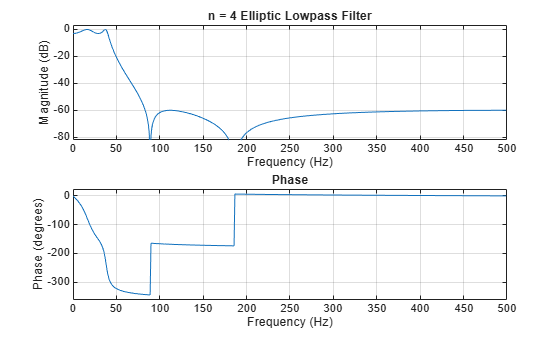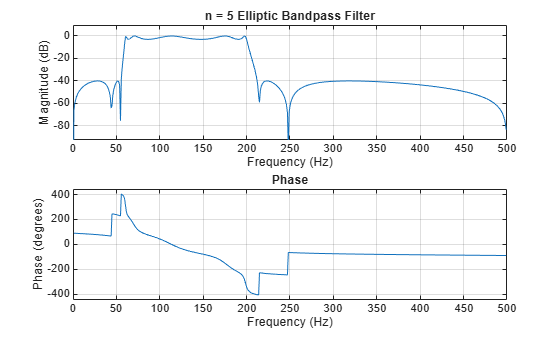Documentation

### This is machine translation

Mouseover text to see original. Click the button below to return to the English version of the page.

# ellipord

Minimum order for elliptic filters

## Syntax

```[n,Wp] = ellipord(Wp,Ws,Rp,Rs) [n,Wp] = ellipord(Wp,Ws,Rp,Rs,'s') ```

## Description

`ellipord` calculates the minimum order of a digital or analog elliptic filter required to meet a set of filter design specifications.

### Digital Domain

`[n,Wp] = ellipord(Wp,Ws,Rp,Rs)` returns the lowest order, `n`, of the elliptic filter that loses no more than `Rp` dB in the passband and has at least `Rs` dB of attenuation in the stopband. The scalar (or vector) of corresponding cutoff frequencies `Wp`, is also returned. Use the output arguments `n` and `Wp` in `ellip`.

Choose the input arguments to specify the stopband and passband according to the following table.

Description of Stopband and Passband Filter Parameters

Parameter

Description

`Wp`

Passband corner frequency `Wp`, the cutoff frequency, is a scalar or a two-element vector with values between 0 and 1, with 1 corresponding to the normalized Nyquist frequency, π radians per sample.

`Ws`

Stopband corner frequency `Ws`, is a scalar or a two-element vector with values between 0 and 1, with 1 corresponding to the normalized Nyquist frequency.

`Rp`

Passband ripple, in decibels. This value is the maximum permissible passband loss in decibels.

`Rs`

Stopband attenuation, in decibels. This value is the number of decibels the stopband is attenuated with respect to the passband response.

Use the following guide to specify filters of different types.

Filter Type Stopband and Passband Specifications

Filter Type

Stopband and Passband Conditions

Stopband

Passband

Lowpass

`Wp` < `Ws`, both scalars

`(Ws,1)`

`(0,Wp)`

Highpass

`Wp` > `Ws`, both scalars

`(0,Ws)`

`(Wp,1)`

Bandpass

The interval specified by `Ws` contains the one specified by `Wp` (```Ws(1) < Wp(1) < Wp(2) < Ws(2)```).

`(0,Ws(1))` and `(Ws(2),1)`

`(Wp(1),Wp(2))`

Bandstop

The interval specified by `Wp` contains the one specified by `Ws` (```Wp(1) < Ws(1) < Ws(2) < Wp(2)```).

`(0,Wp(1))` and `(Wp(2),1)`

`(Ws(1),Ws(2))`

If your filter specifications call for a bandpass or bandstop filter with unequal ripple in each of the passbands or stopbands, design separate lowpass and highpass filters according to the specifications in this table, and cascade the two filters together.

### Analog Domain

`[n,Wp] = ellipord(Wp,Ws,Rp,Rs,'s')` finds the minimum order `n` and cutoff frequencies `Wp` for an analog filter. You specify the frequencies `Wp` and `Ws` similar to those described in the Description of Stopband and Passband Filter Parameters table above, only in this case you specify the frequency in radians per second, and the passband or the stopband can be infinite.

Use `ellipord` for lowpass, highpass, bandpass, and bandstop filters as described in the Filter Type Stopband and Passband Specifications table above.

## Examples

collapse all

For 1000 Hz data, design a lowpass filter with less than 3 dB of ripple in the passband, defined from 0 to 40 Hz, and at least 60 dB of ripple in the stopband, defined from 150 Hz to the Nyquist frequency, 500 Hz. Find the filter order and cutoff frequency.

```Wp = 40/500; Ws = 150/500; Rp = 3; Rs = 60; [n,Wp] = ellipord(Wp,Ws,Rp,Rs)```
```n = 4 ```
```Wp = 0.0800 ```

Specify the filter in terms of second-order sections and plot the frequency response.

```[z,p,k] = ellip(n,Rp,Rs,Wp); sos = zp2sos(z,p,k); freqz(sos,512,1000) title(sprintf('n = %d Elliptic Lowpass Filter',n))```Design a bandpass filter with a passband from 60 Hz to 200 Hz with at most 3 dB of ripple and at least 40 dB attenuation in the stopbands. Specify a sampling rate of 1 kHz. Have the stopbands be 50 Hz wide on both sides of the passband. Find the filter order and cutoff frequencies.

```Wp = [60 200]/500; Ws = [50 250]/500; Rp = 3; Rs = 40; [n,Wp] = ellipord(Wp,Ws,Rp,Rs)```
```n = 5 ```
```Wp = 1×2 0.1200 0.4000 ```

Specify the filter in terms of second-order sections and plot the frequency response.

```[z,p,k] = ellip(n,Rp,Rs,Wp); sos = zp2sos(z,p,k); freqz(sos,512,1000) title(sprintf('n = %d Elliptic Bandpass Filter',n))```## Algorithms

`ellipord` uses the elliptic lowpass filter order prediction formula described in . The function performs its calculations in the analog domain for both the analog and digital cases. For the digital case, it converts the frequency parameters to the s-domain before estimating the order and natural frequencies, and then converts them back to the z-domain.

`ellipord` initially develops a lowpass filter prototype by transforming the passband frequencies of the desired filter to 1 rad/s (for low- and highpass filters) and to -1 and 1 rad/s (for bandpass and bandstop filters). It then computes the minimum order required for a lowpass filter to meet the stopband specification.

## References

 Rabiner, Lawrence R., and B. Gold. Theory and Application of Digital Signal Processing. Englewood Cliffs, NJ: Prentice-Hall, 1975.